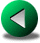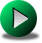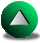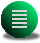Previous: Conclusions
Next: Model vorticity budget on
Up: No Title

# an A-grid energy conserving formulation

Table A.1: Notations for the finite volume method
 i cell index Voli cell Volume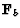normal oriented face lengthany variable interpolated at the center of face neigh face index for the i-cell

It is possible to formulate an energy conserving scheme on a A-grid and generalize it to a finite volume formulation (i.e., irregular domains). We will use the notations of the latter (Table A.1). The time integration has to be done through an iteration process since the formulation is semi-implicit in time, including the non-linear terms.(7.1)(7.2)

where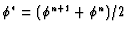for any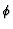. By multiplying A.1 by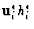, we get(7.3)

Let us define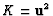and use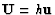, then A.3 reads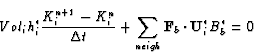(7.4)

and let us multiply A.2 by K*i and use the equivalence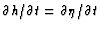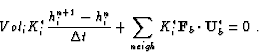(7.5)

We then sum together A.4 and A.5 in order to get an equation for the kinetic energy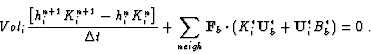(7.6)

The equation for the potential energy is given by multiplying A.2 by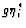(7.7)

Let us define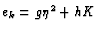. We then get the total energy equation by summing A.6 and A.7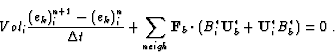(7.8)

Therefore, the total energy budget is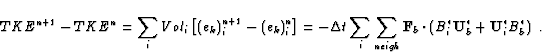(7.9)

Hence, the conservation properties of this scheme comes from the assumption about, the way we interpolate the data onto the faces of the cells. The usual assumption is to take for any,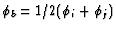where j is the index of the neighboring cell. Because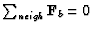, the right hand side simplifies to(7.10)

The right hand side vanishes for open domains. For closed domains, some assumptions are required. If we imagine a fictitious cell on the other side of the wall, we must have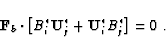(7.11)

This can be satisfied if B*j = B*i. We are then left with satisfying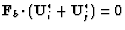. This corresponds to enforcing that the velocity is tangential at the wall. This is a very reasonable assumption since it matches the inviscid boundary condition. Hence, the energy can be conserved for an A-grid scheme in absence of dissipation processes and forcing.

In practice, this scheme only retards the upcoming of spurious modes. In order to control the spurious modes, one idea would be to make the scheme also conserve the enstrophy. According to Abramopoulos abramo88, this is achievable but a unreasonable price.Previous: Conclusions
Next: Model vorticity budget on
Up: No Title

Frederic Dupont
2001-09-11